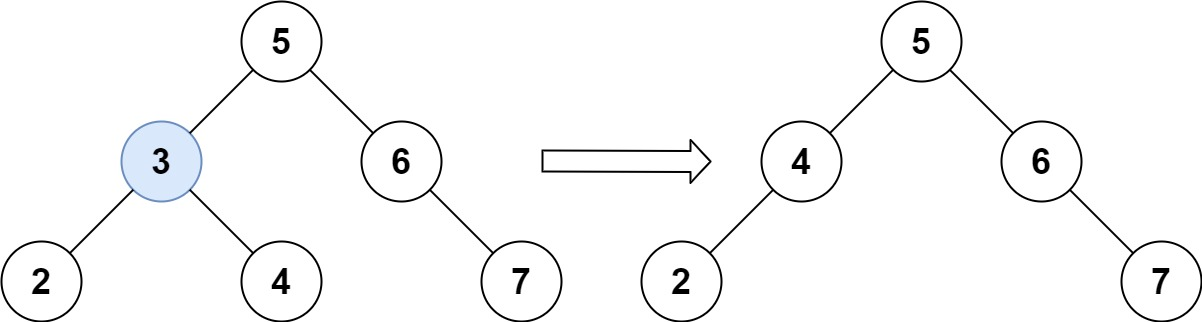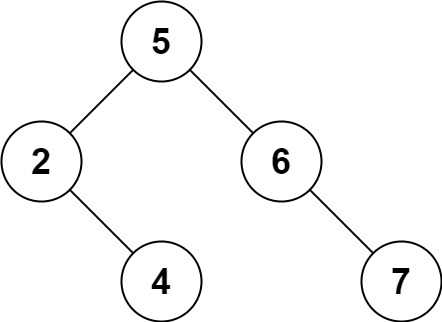## 450. Delete Node in a BST

Given a root node reference of a BST and a key, delete the node with the given key in the BST. Return the root node reference (possibly updated) of the BST.

Basically, the deletion can be divided into two stages:

1. Search for a node to remove.
2. If the node is found, delete the node.

Follow up: Can you solve it with time complexity `O(height of tree)`?

Example 1:```Input: root = [5,3,6,2,4,null,7], key = 3
Output: [5,4,6,2,null,null,7]
Explanation: Given key to delete is 3. So we find the node with value 3 and delete it.
One valid answer is [5,4,6,2,null,null,7], shown in the above BST.
Please notice that another valid answer is [5,2,6,null,4,null,7] and it's also accepted.```

Example 2:

```Input: root = [5,3,6,2,4,null,7], key = 0
Output: [5,3,6,2,4,null,7]
Explanation: The tree does not contain a node with value = 0.
```

Example 3:

```Input: root = [], key = 0
Output: []
```

Constraints:

• The number of nodes in the tree is in the range `[0, 104]`.
• `-105 <= Node.val <= 105`
• Each node has a unique value.
• `root` is a valid binary search tree.
• `-105 <= key <= 105`

## Rust Solution

``````struct Solution;
use rustgym_util::*;
use std::cmp::Ordering::*;

trait Delete {
fn delete(self, key: i32) -> TreeLink;
fn smallest(&self) -> i32;
}

impl Delete for TreeLink {
fn delete(self, key: i32) -> TreeLink {
if let Some(link) = self {
let mut node = link.borrow_mut();
let left = node.left.take();
let right = node.right.take();
let val = node.val;
match key.cmp(&val) {
Equal => match (left, right) {
(None, None) => None,
(Some(left), None) => Some(left),
(None, Some(right)) => Some(right),
(left, right) => {
let smallest = right.smallest();
tree!(smallest, left, right.delete(smallest))
}
},
Less => tree!(val, left.delete(key), right),
Greater => tree!(val, left, right.delete(key)),
}
} else {
None
}
}
fn smallest(&self) -> i32 {
if let Some(node) = self {
let node = node.borrow();
let val = node.val;
if node.left.is_some() {
node.left.smallest()
} else {
val
}
} else {
unreachable!()
}
}
}

impl Solution {
fn delete_node(root: TreeLink, key: i32) -> TreeLink {
root.delete(key)
}
}

#[test]
fn test() {
let root = tree!(5, tree!(3, tree!(2), tree!(4)), tree!(6, None, tree!(7)));
let key = 3;
let res = tree!(5, tree!(4, tree!(2), None), tree!(6, None, tree!(7)));
assert_eq!(Solution::delete_node(root, key), res);
}
``````

Having problems with this solution? Click here to submit an issue on github.